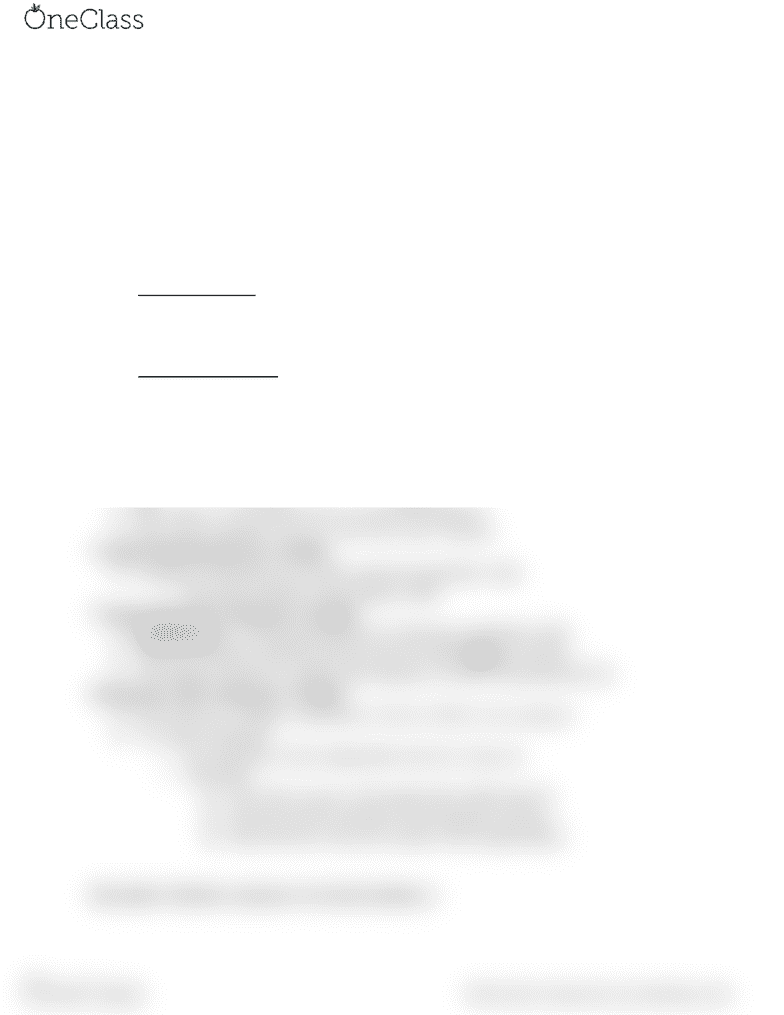# APST 207 Lecture 3: Frequency Distributions:Cental Tendency

23 views2 pages
School
Course

For unlimited access to Class Notes, a Class+ subscription is required.Frequency Distributions
Quantitative distributions and histograms
Quantitative Variables
Represent a measurable quantity
Numerical
Number of credits taken fall semester
Pumpkin spice latte likability
Can be meaningfully averaged,multiplied, divided and subtracted
Discrete vs Continuous
Discrete variables
Countable number of value
Whole numbers- can you count it on your fingers ?
Examples: # of students in a class or correct answers on a tes
Continuous variables
The unit of measure is divisible-a case could have any value in the unit
We can have decimals
Examples: Snowfall on Mt. Rose, GPA
Discrete or Continuous
How many hipsters are in Bibo’s ? discrete
What was your grade percentage on the first lab ? continuous
How many cc’s of solution are in a syringe? Continuous
How many accidents occur on 1-80 every week ? discrete
Visually Depicting discrete variables
Frequency distributions are used to represent quantitative data
The easiest method is the frequency table
Visually Depicting Continuous Variables
Histograms are visual representations of continuous quantitative data
They are created by defining discrete intervals called bins (or classes)
The height of each bin is equal to the frequency of cases in that particular bin
Histograms with Continuous Variables
Given that our variable is continuous we have to find a way to group it
To create a histogram
We create bins with an appropriate interval or step size
About bins:
The value you give is the upper limit of that interval
The bins must be exhaustive and mutually exclusive
Place the bins in the next column with a header (Bins)
Descriptive Statistics (measures of central tendency )
find more resources at oneclass.com
find more resources at oneclass.com
Unlock document

This preview shows half of the first page of the document.
Unlock all 2 pages and 3 million more documents.

Already have an account? Log in

# Get access

Grade+
\$10 USD/m
Billed \$120 USD annually
Homework Help
Class Notes
Textbook Notes
40 Verified Answers
Study Guides
1 Booster Class
Class+
\$8 USD/m
Billed \$96 USD annually
Homework Help
Class Notes
Textbook Notes
30 Verified Answers
Study Guides
1 Booster Class